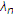# Recurring Mean Inequality of Random Variables

## Abstract

A multidimensional recurring mean inequality is shown. Furthermore, we prove some new inequalities, which can be considered to be the extensions of those established inequalities, including, for example, the Polya-Szegö and Kantorovich inequalities.

## 1. Introduction

The theory of means and their inequalities is fundamental to many fields including mathematics, statistics, physics, and economics.This is certainly true in the area of probability and statistics. There are large amounts of work available in the literature. For example, some useful results have been given by Shaked and Tong , Shaked and Shanthikumar , Shaked et al. , and Tong [4, 5]. Motivated by different concerns, there are numerous ways to introduce mean values. In probability and statistics, the most commonly used mean is expectation. In , the author proves the mean inequality of two random variables. The purpose of the present paper is to establish a recurring mean inequality, which generalizes the mean inequality of two random variables torandom variables. This result can, in turn, be extended to establish other new inequalities, which include generalizations of the Polya-Szegö and Kantorovich inequalities .

We begin by introducing some preliminary concepts and known results which can also be found in .

Definition 1.1.

The supremum and infimum of the random variableare defined asand, respectively, and denoted byand.

Definition 1.2.

Ifis bounded, the arithmetic mean of the random variable,, is given by(1.1)

In addition, if, one defines the geometric mean of the random variable,, to be(1.2)

Definition 1.3.

Ifare bounded random variables, the independent arithmetic mean of the product of random variablesis given by(1.3)

Definition 1.4.

Ifare bounded random variables with, one defines the independent geometric mean of the product of random variablesto be(1.4)

Remark 1.5.

Ifare independent, then(1.5)

The mean inequality of two random variables .

Theorem 1.6.

Letandbe bounded random variables. Ifand, then(1.6)

Equality holds if and only if(1.7)

for.

## 2. Main Results

Our main results are given by the following theorem.

Theorem 2.1.

Suppose thatare bounded random variables,. Letbe a sequence of real numbers. If(2.1)

then(2.2)

Proof.

Let. We have(2.3)

So(2.4)

which implies that(2.5)

Using the Jensen inequality  and assumption (2.1), we get(2.6)

Hence,(2.7)

from which (2.2) follows.

Combining this result with Theorem 1.6, the following recurring inequalities are immediate.

Corollary 2.2.

Letbe bounded random variables. If,, then(2.8)

## 3. Some Applications

In this section, we exhibit some of the applications of the inequalities just obtained. We make use of the following known lemma which we state here without proof.

Lemma 3.1.

If, then(3.1)

Theorem 3.2 (the extensions of the inequality of Polya-Szegö).

Let, forand. Then,(3.2)

Proof.

This result is a consequence of inequality (2.8). Lethave the distribution(3.3)

We definefunctions as follows:(3.4)

Let. Then,(3.5)

Inequality (2.8) then becomes(3.6)

from which our result follows.

Remark 3.3.

For, we can get the inequality of Polya-Szegö :(3.7)

where, and.

Theorem 3.4 (the extensions of Kantorovich's inequality).

Letbe anpositive Hermitian matrix. Denote byandthe maximum and minimum eigenvalues of, respectively. For realand, and any vector,the following inequality is satisfied:(3.8)

where(3.9)

Proof.

Letbe eigenvalues ofand let. There is a Hermitian matrixthat satisfies(3.10)

Let(3.11)

Then,(3.12)

What remains to show is that(3.13)

We define the random variable, and assign. SupposeNotice thatandare the upper and lower bounds of the random variable, soandare the lower and upper bounds of. According to Lemma 3.1, we know that(3.14)

Noticing that(3.15)

we can use inequality (2.8) to express inequality (3.13) as(3.16)

Remark 3.5.

Ifand, this inequality takes the form(3.17)

which is Kantorovich's inequality .

## References

1. Shaked M, Tong YL: Inequalities for probability contents of convex sets via geometric average. Journal of Multivariate Analysis 1988,24(2):330–340. 10.1016/0047-259X(88)90043-7

2. Shaked M, Shanthikumar JG: Stochastic Orders and Their Applications, Probability and Mathematical Statistics. Academic Press, Boston, Mass, USA; 1994:xvi+545.

3. Shaked M, Shanthikumar JG, Tong YL: Parametric Schur convexity and arrangement monotonicity properties of partial sums. Journal of Multivariate Analysis 1995,53(2):293–310. 10.1006/jmva.1995.1038

4. Tong YL: Some recent developments on majorization inequalities in probability and statistics. Linear Algebra and Its Applications 1994,199(supplement 1):69–90.

5. Tong YL: Relationship between stochastic inequalities and some classical mathematical inequalities. Journal of Inequalities and Applications 1997,1(1):85–98. 10.1155/S1025583497000064

6. Wang M: The mean inequality of random variables. Mathematical Inequalities & Applications 2002,5(4):755–763.

7. Hardy GH, Littlewood JE, Pólya G: Inequalities. 2nd edition. Cambridge University Press, Cambridge, UK; 1952.

## Author information

Authors

### Corresponding author

Correspondence to Mingjin Wang.

## Rights and permissions

Reprints and Permissions

Wang, M. Recurring Mean Inequality of Random Variables. J Inequal Appl 2008, 325845 (2008). https://doi.org/10.1155/2008/325845

• Revised:

• Accepted:

• Published:

• DOI: https://doi.org/10.1155/2008/325845

### Keywords

• Real Number
• Lower Bound
• Hermitian Matrix
• Minimum Eigenvalue
• Jensen Inequality